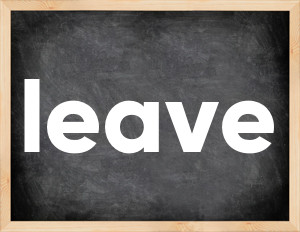# Leave past tenseThe English verb 'leave' is pronounced as [li:v].
Related to: irregular verbs.
3 forms of verb leave: Infinitive (leave), Past Simple - (left), Past Participle - (left).

## Here are the past tense forms of the verb leave

👉 Forms of verb leave in future and past simple and past participle.
❓ What is the past tense of leave.

## Leave: Past, Present, and Participle Forms

Base Form Past Simple Past Participle
leave [li:v]

left [lɛft]

left [lɛft]

## What are the 2nd and 3rd forms of the verb leave?

🎓 What are the past simple, future simple, present perfect, past perfect, and future perfect forms of the base form (infinitive) 'leave'?

### Learn the three forms of the English verb 'leave'

• the first form (V1) is 'leave' used in present simple and future simple tenses.
• the second form (V2) is 'left' used in past simple tense.
• the third form (V3) is 'left' used in present perfect and past perfect tenses.

## What are the past tense and past participle of leave?

The past tense and past participle of leave are: leave in past simple is left, and past participle is left.

### What is the past tense of leave?

The past tense of the verb "leave" is "left", and the past participle is "left".

### Verb Tenses

Past simple — leave in past simple left (V2).
Future simple — leave in future simple is leave (will + V1).
Present Perfect — leave in present perfect tense is left (have/has + V3).
Past Perfect — leave in past perfect tense is left (had + V3).

### leave regular or irregular verb?

👉 Is 'leave' a regular or irregular verb? The verb 'leave' is irregular verb.

## Examples of Verb leave in Sentences

•   John, we can't leave them like this (Present Simple)
•   They know you left your firm three weeks ago (Past Simple)
•   It has left 4 million children orphaned (Present Perfect)
•   He is leaving now, you don't have time to say goodye (Present Continuous)
•   When he was leaving it started to rain (Past Continuous)
•   They usually leave home at 8.30 (Present Simple)
•   If you don't leave me now, I will call the police (Present Simple)
•   He left nothing after passing away (Past Simple)